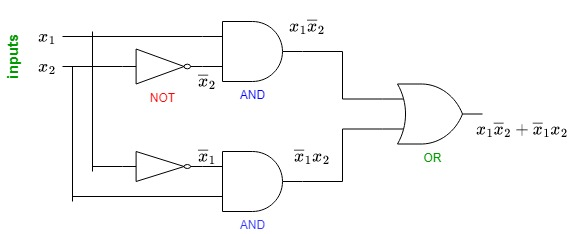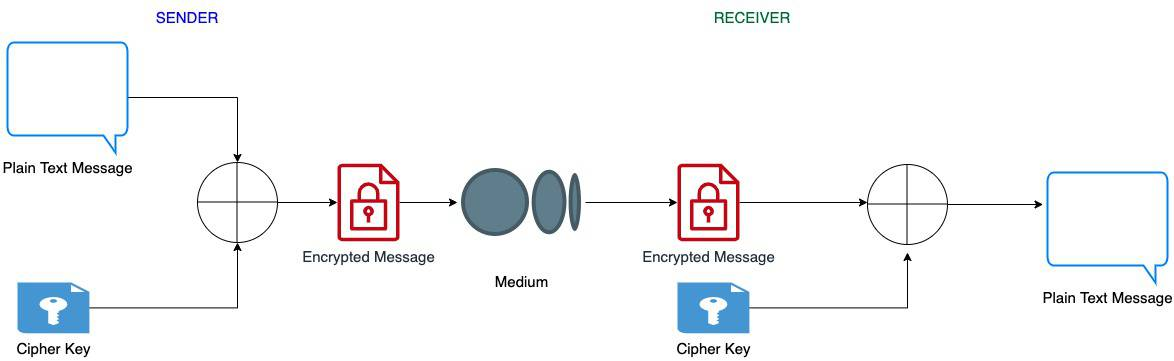## 1. Introduction

In this tutorial, we’ll study the exclusive OR operation, which is also called XOR.

XOR is widely used in many fields such as cryptography, genetic algorithms, and digital signal processing.

## 2. Bit Operations

XOR is a bit operation. Bit operations are mathematical functions we perform on individual bits of input numbers. They are very fast and robust because they use fewer CPU cycles and are carried out in only one CPU register. In contrast, most arithmetic instructions use more than one register, so they spend more time moving the arguments and intermediate results between the registers.

There are two types of bit operations in computer programming: unary and binary.

Unary operations take only one input. NOT is one of the most popular unary operators. It negates the input bit, so if, then, and vice versa.

Binary operations take two inputs. OR, AND and XOR are some of the most popular binary bit operations.  For instance, AND gives as output 1 if both the operands have their bit set as 1, else it gives as output 0. So, ifand, then.

## 3. XOR Operation

XOR accepts two operands and compares their values. If they’re the same, it evaluates to 0 (or False). Otherwise, if both operands have different values, it outputs 1 (or True). Here’s its truth table:### 3.1. Hardware Implementation

The following figure shows the internal implementation of the XOR operation at the hardware level:As we see, hardware uses 5 gates (2 ANDs, 2 NOTs, and one OR) to implement XOR, whereandare our input bits.

Firstly, we feedto one NOT gate. Its output along withthen flows to the first AND gate. Similarly, we feedto the other NOT gate and forward its output along withto the second AND gate. The outputs of both AND gates are then fed to the OR gate.

We get the result of the XOR operation for the inputsandas the output of the OR gate.

Why do we implement XOR like this? If we look at its truth table, we’ll see that this implementation enumerates the cases where XOR is True:and.

### 4. XOR in Cryptography

In this section, we explore the use of XOR in cryptography.

### 4.1. XOR in Encryption Schemes

We can use XOR in two-way encryption algorithms that are executed at both the sender’s and the receiver’s ends. The sender and the receiver both have the same cipher key. The sender uses this key to encrypt the message and send it to the receiver. The receiver gets this encoded message and decrypts it with the same to get the original message back.

The following diagram depicts this flow:### 4.2. Example

Let’s suppose our cipher keyis 11001001 and our messageis 11100101. We apply XOR toandto get an encoded message:On the receiver side, we apply XOR toandto get our original messageback:### 4.3. Advantages of XOR in Cryptography

XOR offers two big advantages here. First, XOR doesn’t leak information about the original message. Why? Well, if, we cannot be sure about the exact values ofand. They can be either (1, 0) or (0, 1). The same goes if the result is 0: the arguments can be either (1, 1) or (0, 0).

In contrast, AND and OR leak information. If, we know that. If, we can be sure that.

Secondly, XOR is an involutory function. Formally, a functionis involutory ifwheneveris defined. Thus, if we apply XOR twice, we’ll get the original plain text back. In other words:The inner XOR acts as the encryption operation whereas the outer XOR decrypts the message.

## 5. XOR Use Cases

There are many things we can use XOR for. For instance, to generate parity bits for error checking and fault tolerance, or to swap two values without auxiliary storage.

### 5.1. Swapping Without Using XOR

Traditionally, we swap the values of two variables using temporary storage as shown in the following pseudocode:This way, we need additional storage for the temporary variable temp.

### 5.2. Swapping Using XOR

Now, let’s use the XOR operation to swap two values. As shown in the peudocode, we don’t use any additional storage:freestar.config.enabled_slots.push({ placementName: "baeldung_incontent_2", slotId: "baeldung_incontent_2" });



Does this work? Let’s check.

Let’s say that, initially,and. In the binary representation,and.

Now, the first XOR updates the value of:Then, by applying XOR toand the new value of, we recover the oldand assign the result to:Finally, using the updated value offrom the above step, the third XOR recovers the initial value of, so we assign it to:In the decimal representation, we getand. As we see, the values have been swapped, which is what we wanted to achieve.

## 6. Conclusion

In this article, we studied the XOR operation in computer programming. XOR is used in many encryption algorithms because it doesn’t leak information and can recover the original message after encryption. Further, XOR is faster than most arithmetic operations as it runs fewer instructions and mostly uses a single CPU register for execution.